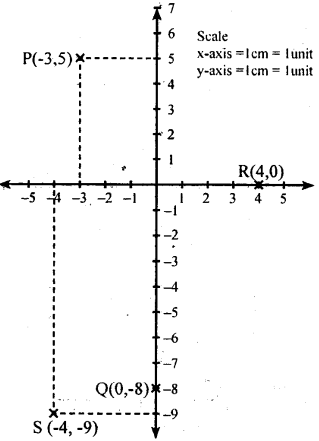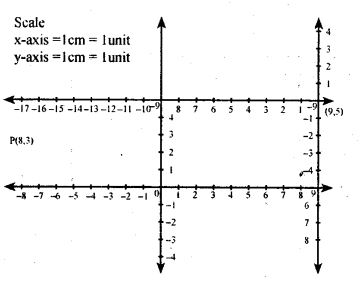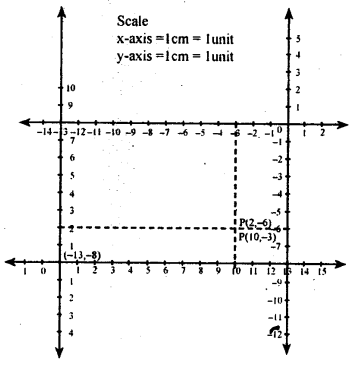# KSEEB Solutions for Class 8 Maths Chapter 14 Introduction of Graphs Ex 14.1

In this chapter, we provide KSEEB SSLC Class 8 Maths Chapter 14 Introduction of Graphs Ex 14.1 for English medium students, Which will very helpful for every student in their exams. Students can download the latest KSEEB SSLC Class 8 Maths Chapter 14 Introduction of Graphs Ex 14.1 pdf, free KSEEB SSLC Class 8 Maths Chapter 14 Introduction of Graphs Ex 14.1 pdf download. Now you will get step by step solution to each question.

### Karnataka State Syllabus Class 8 Maths Chapter 14 Introduction of Graphs Ex 14.1

Question 1.
Fix up your own coordinate system on a graph paper and locate the following points on the sheet,
i. P(-3,5)
ii. Q(0,-8)
iii. R(4,0)
iv. S (-4, -9)Question 2.
Suppose you are given a coordinate system to determine the quadrant in which the following points lie.
i. A (4, 5) – I Quadrant
ii. B (-4, -5) – III Quadrant
iii. C (4, -5) – IV Quadrant

Question 3.
Suppose p is a point with coordinate (-8, 3) with respect to a coordinate system XO X → YOY. Let X11 O1 X1 ↔ Y1OY be another system with X1OX  ||  X11O1X1 and O1 has coordinates (9,5) with respect to X1OX ↔ Y1O Ywhat are the coordinate of p in the system X11 O1X1 Y11O1Y1?
Let (x,y1) be the coordinates point p in X11 O1 X1 ↔ Y1OY.
Then x = a + xand y = b + y1
but (a, b) = (9, 5)
∴ – 8 = 9 +x1      y = b + y1
– 8 – 9 = x1         3 = 5 + y1
-17 = x1             3 – 5 = y1
∴ (x1 , y1 ) = (-17,-2)
∴ Coordinatesof pin X11 O1 X1 ↔ Y1OY are (-17,-2)Question 4.
Suppose P has coordinates (10,2) in a coordinate system X’OX ↔ Y’OY and (-3,-6) in an other coordinates system X’1OX↔Y’1O1X1. Determine the coordinates of O with respect to the system X’1 O1 X|| Y’1 O1Y1’.
Given p(x , y) = (10 , 2), (x’, y’) = (-3, -6)
Let the coordinates of O, in
X’1O1 X1 ↔ Y’1O1 Y1 be (a,b) then
x = a + x1           y = b + y1
10 = a + (-3)     2 = b + (-6)
10 = a – 3          2 = b – 6
10 + 3 = a       2 + 6 = b
a = 13                    b = 8
Coordinates of O1 in
X’1 O 1Y1, ↔ Y1 O 1Y’ is (13 , 8) we should find the coordinates of O
(0, 0) in X’11X1↔ Y’1O1Y1
Let (x , y) = (0 , 0) , (x’, y’) = (13 , 8) .
(a ,b) = ?            y = b + y’
0 = a + 13              0 = b + 8
-13 = a                    -8 = b
(a = – 13)                      b= -8
The coordinates of O w.r.t to
X’1 O 1Y1, ↔ Y1 O 1Y’1 are (-13 , -8).All Chapter KSEEB Solutions For Class 8 maths

—————————————————————————–

All Subject KSEEB Solutions For Class 8

*************************************************

I think you got complete solutions for this chapter. If You have any queries regarding this chapter, please comment on the below section our subject teacher will answer you. We tried our best to give complete solutions so you got good marks in your exam.

If these solutions have helped you, you can also share kseebsolutionsfor.com to your friends.

Best of Luck!!